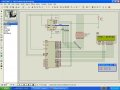# RTC12C887 Interfacing Error

#### scintrillating

Joined Dec 30, 2011
5
Guys...I am making a Real Time Clock using DS12c887 and 16X2 LCD...
I following code works great to display seconds extracted from the RTC on the LCD but hours and minutes are not displayed in the way it should. They take any random value...Please find out the solution to it and suggest some debugging techniques...

Try to simulate this using Proteus and you will see the error!

Rich (BB code):
#include <REGX51.H>
#include<ABSACC.h>

#define port P3
#define lcd_dataport P2  // lcd_dataport for lcd
#define timeport P0 // Port for RTC

sbit rs = port^0;
sbit rw = port^1;
sbit en = port^2;

int hr, min, sec;

void delay(unsigned int msec) //Time delay function
{
int i,j ;
for(i=0;i<msec;i++)
for(j=0;j<1275;j++);
}

void lcdcmd(unsigned char item) //Function to send command to LCD
{
lcd_dataport = item;
rs= 0;
rw=0;
en=1;
delay(1);
en=0;
return;
}

void lcddata(unsigned char item) //Funtion to send data on LCD
{
lcd_dataport = item;
rs= 1;
rw=0;
en=1;
delay(1);
en=0;
}

void lcddata_string(unsigned char *str) // Function to send string on LCD
{
int i=0;
while(str!='\0')
{
lcddata(str);
i++;
delay(1);
}
}

void lcd(unsigned char str)  // Funtion to Initialize LCD
{
lcdcmd(0x38);
lcdcmd(0x0C);
lcdcmd(0x01);
lcdcmd(0x80);
lcddata_string(str);
}

void check_key()
{
if(key0==0)
lcd("SET TIME");
if(key1==0)
lcd("Increment");
if(key2==0)
lcd("Decrement");
if(key3==0)
lcd("SET A");
if(key4==0)
lcd("SET B");
if(key5==0)
lcd("SET C");
if(key6==0)
lcd("SET BANGS");
if(key7==0)
lcd("SET GROUPS");
}

void bcdconv(unsigned char mybyte)
{
unsigned char x,y;
x=mybyte&0x0F;
x=x|0x30;
y=mybyte&0xF0;
y=y>>4;
y=y|0x30;
lcddata(y);
lcddata(x);
}

void main()
{
delay(220);
XBYTE=0x20;
XBYTE=0x82;
XBYTE=0x16;
XBYTE=0x56;
XBYTE=0x41;
XBYTE=0x02;
lcd("TIME:");
while(1)
{
lcdcmd(0x88);
hr=XBYTE;
bcdconv(hr);
lcddata(':');
min=XBYTE;
bcdconv(min);
lcddata(':');
sec=XBYTE;
bcdconv(sec);
}
}



Here is the screenshot of the Proteus simulated circuitLast edited by a moderator:

#### SgtWookie

Joined Jul 17, 2007
22,221
You know, I don't see anyplace else but the #defines where you reference either timeport or P0. Since you are not reading from or writing to the time port, why did you think you might get some time data in your program?

[eta]
I have gone to the trouble to re-indent your C code the way it should be. If you don't keep your code indented properly, you may omit a curly brace somewhere.

Rich (BB code):
#include <REGX51.H>
#include<ABSACC.h>

#define port P3
#define lcd_dataport P2  // lcd_dataport for lcd
#define timeport P0      // Port for RTC

sbit rs = port^0;
sbit rw = port^1;
sbit en = port^2;

int hr, min, sec;

void delay(unsigned int msec) //Time delay function
{
int i,j ;
for(i=0;i<msec;i++)
for(j=0;j<1275;j++);
}

void lcdcmd(unsigned char item) //Function to send command to LCD
{
lcd_dataport = item;
rs= 0;
rw=0;
en=1;
delay(1);
en=0;
return;
}

void lcddata(unsigned char item) //Funtion to send data on LCD
{
lcd_dataport = item;
rs= 1;
rw=0;
en=1;
delay(1);
en=0;
}

void lcddata_string(unsigned char *str) // Function to send string on LCD
{
int i=0;
while(str!='\0')
{
lcddata(str);
i++;
delay(1);
}
}

void lcd(unsigned char str)  // Funtion to Initialize LCD
{
lcdcmd(0x38);
lcdcmd(0x0C);
lcdcmd(0x01);
lcdcmd(0x80);
lcddata_string(str);
}

void check_key()
{
if(key0==0)
lcd("SET TIME");
if(key1==0)
lcd("Increment");
if(key2==0)
lcd("Decrement");
if(key3==0)
lcd("SET A");
if(key4==0)
lcd("SET B");
if(key5==0)
lcd("SET C");
if(key6==0)
lcd("SET BANGS");
if(key7==0)
lcd("SET GROUPS");
}

void bcdconv(unsigned char mybyte)
{
unsigned char x,y;
x=mybyte&0x0F;
x=x|0x30;
y=mybyte&0xF0;
y=y>>4;
y=y|0x30;
lcddata(y);
lcddata(x);
}

void main()
{
delay(220);
XBYTE=0x20;
XBYTE=0x82;
XBYTE=0x16;
XBYTE=0x56;
XBYTE=0x41;
XBYTE=0x02;
lcd("TIME:");
while(1)
{
lcdcmd(0x88);
hr=XBYTE;
bcdconv(hr);
lcddata(':');
min=XBYTE;
bcdconv(min);
lcddata(':');
sec=XBYTE;
bcdconv(sec);
}
}


Last edited:

#### Santosh_16k

Joined Aug 19, 2011
19
Shrimohan Jhawar, I have the same problem!